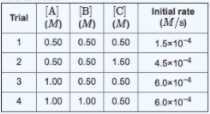# Problem: For the reaction A + B + C → D + E, the initial reaction rate was measured for various initial concentrations of reactants. The following data were collected: What is the value of the rate constant k for this reaction? When entering compound units, indicate multiplication of units explicitly using a multiplication dot (in the menu). For example, M-1 • s-1.Express your answer to two significant figures and include the appropriate units. Indicate the multiplication of units explicitly either with a multiplication dot or a dash.

###### FREE Expert Solution
85% (488 ratings)
###### FREE Expert Solution
85% (488 ratings)###### Problem Details

For the reaction A + B + C → D + E, the initial reaction rate was measured for various initial concentrations of reactants. The following data were collected:

What is the value of the rate constant k for this reaction?

When entering compound units, indicate multiplication of units explicitly using a multiplication dot (in the menu). For example, M-1 • s-1.

Express your answer to two significant figures and include the appropriate units. Indicate the multiplication of units explicitly either with a multiplication dot or a dash.